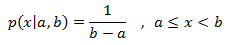class template
<random>

# std::uniform_real_distribution

`template <class RealType = double> class uniform_real_distribution;`
Uniform real distribution
Random number distribution that produces floating-point values according to a uniform distribution, which is described by the following probability density function:This distribution (also know as rectangular distribution) produces random numbers in a range [a,b) where all intervals of the same length within it are equally probable.

The distribution parameters, a and b, are set on construction.

To produce a random value following this distribution, call its member function operator().

### Template parameters

RealType
A floating-point type. Aliased as member type result_type.
By default, this is double.

### Member types

The following aliases are member types of uniform_real_distribution:

member typedefinitionnotes
result_typeThe first template parameter (RealType)The type of the numbers generated (defaults to double)
param_typenot specifiedThe type returned by member param.

### Example

 ``123456789101112131415161718192021222324252627282930`` ``````// uniform_real_distribution #include #include int main() { const int nrolls=10000; // number of experiments const int nstars=95; // maximum number of stars to distribute const int nintervals=10; // number of intervals std::default_random_engine generator; std::uniform_real_distribution distribution(0.0,1.0); int p[nintervals]={}; for (int i=0; i

Possible output:
 ```uniform_real_distribution (0.0,1.0): 0.0-0.1: ********* 0.1-0.2: ********* 0.2-0.3: ********* 0.3-0.4: ********* 0.4-0.5: ********* 0.5-0.6: ********* 0.6-0.7: ********* 0.7-0.8: ********* 0.8-0.9: ********* 0.9-1.0: ********* ```